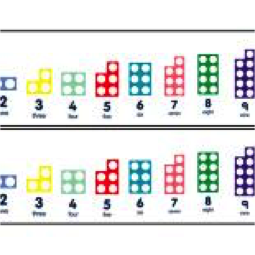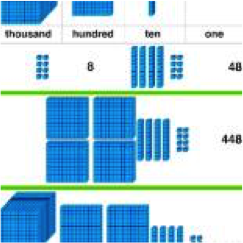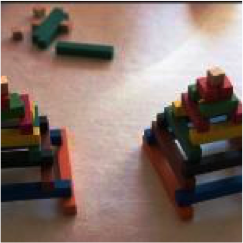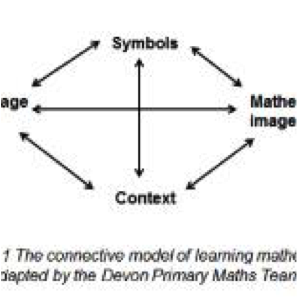# Maths

At South Molton United C of E Primary School, we believe in supporting children with their mental mathematics alongside developing a deep understanding of the four operations (addition, subtraction, multiplication and division) using written recordings.

A range of mental strategies will help them to understand number and calculate an unknown with speed and confidence. When they show a deep understanding of place value and the operations, we teach formal written methods for calculations that cannot be done mentally. Children should be exposed to a wide range of real life problems to solve, so that they can see the variety of different strategies there are and begin to choose the best strategy independently. When calculating, children should estimate and check to ensure accuracy. During assessments, children must write the calculation as a number sentence and ensure their workings are clear for someone else to understand.

It is vital that children develop a strong and secure conceptual understanding of the four operations. Therefore we use apparatus such as NUMICON, Base 10, Cuisenaire and other relevant resources to support the development of this understanding. We also use the connective model of maths so that children understand the language, the images and models, the strategies and how to apply them for each operation.It is important that children are able to apply the most efficient and suitable method for a given calculation and that they think like mathematicians, choosing and applying the best strategies and estimating prior to calculating. They should also check their calculations using the inverse.

The New National Curriculum for Mathematics aims to ensure that all pupils:

• become fluent in the fundamentals of mathematics, including through varied and frequent practice with increasingly complex problems over time, so that pupils develop conceptual understanding and the ability to recall and apply knowledge rapidly and accurately.
• reason mathematically by following a line of enquiry, conjecturing relationships and generalisations, and developing an argument, justification or proof using mathematical language.
• can solve problems by applying their mathematics to a variety of routine and non-routine problems with increasing sophistication, including breaking down problems into a series of simpler steps and persevering in seeking solutions.
• At South Molton United C of E Primary, we use the Rising Stars planning documents to ensure progression throughout all year groups. This framework has been written to provide teachers with a structure for designing learning  sequences covering the content of the National Curriculum and is underpinned by its aims, namely that all pupils should:

★ solve problems

★ reason mathematically

★ become fluent in the fundamentals of mathematics.

The sequences visited each term are Number Sense, Additive Reasoning, Multiplicative Reasoning, Geometric reasoning

Number Sense – this theme is about understanding our number system, starting with counting numbers and building on this understanding to include negative numbers, fractions and decimals.

Additive Reasoning – this theme is about all aspects of understanding addition and subtraction.Multiplicative Reasoning – this theme is about all aspects of understanding multiplication and division including fractions.

Geometric Reasoning – this theme is about all aspects of understanding shape and space and includes application of understanding of number and calculation.

Calculations Policy